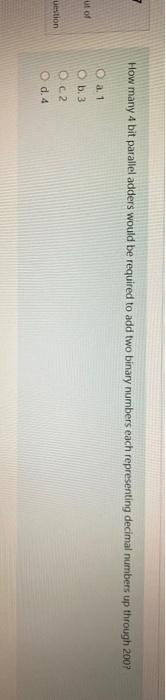# Question How many 4 bit parallel adders would be required to add two binary numbers each representing decimal numbers up through 2007 ut of O a. 1 O b. 3 uestion Oca O d. 4EFDPYN The Asker · Electrical EngineeringTranscribed Image Text: How many 4 bit parallel adders would be required to add two binary numbers each representing decimal numbers up through 2007 ut of O a. 1 O b. 3 uestion Oca O d. 4
More
Transcribed Image Text: How many 4 bit parallel adders would be required to add two binary numbers each representing decimal numbers up through 2007 ut of O a. 1 O b. 3 uestion Oca O d. 4